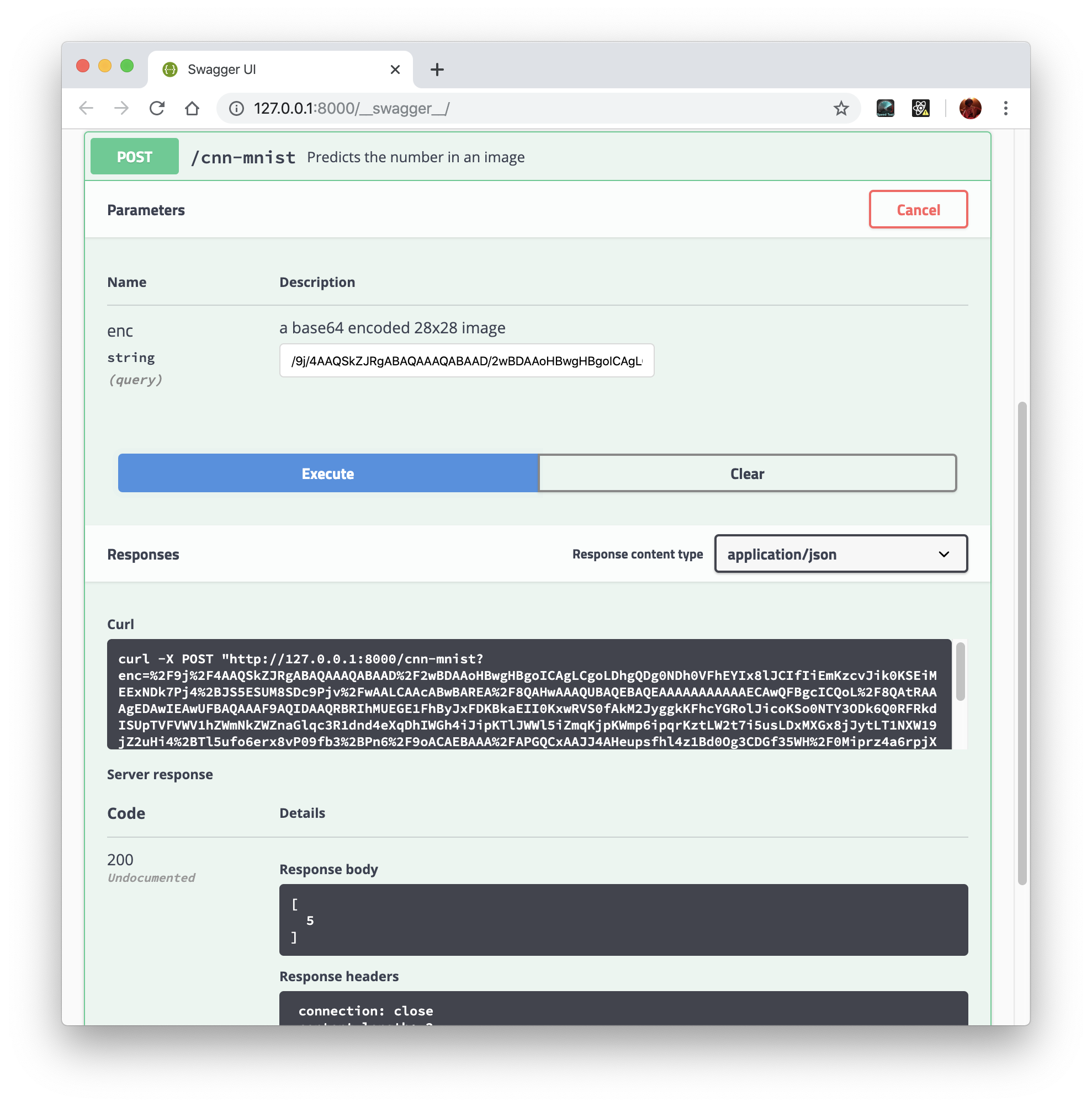# Plumber API

In this tutorial you will learn how to deploy a TensorFlow model using a plumber API.

In this example we will build an endpoint that takes `POST` requests sending images containing handwritten digits and returning the predicted number.

## Building the model

The first thing we are going to do is to build our model. W We will use the Keras API to build this model.

We will use the MNIST dataset to build our model.

``````library(keras)
library(tensorflow)
mnist <- dataset_mnist()

mnist\$train\$x <- (mnist\$train\$x/255) %>%
array_reshape(., dim = c(dim(.), 1))

mnist\$test\$x <- (mnist\$test\$x/255) %>%
array_reshape(., dim = c(dim(.), 1))``````

Now, we are going to define our Keras model, it will be a simple convolutional neural network.

``````model <- keras_model_sequential() %>%
layer_conv_2d(filters = 16, kernel_size = c(3,3), activation = "relu") %>%
layer_max_pooling_2d(pool_size = c(2,2)) %>%
layer_conv_2d(filters = 16, kernel_size = c(3,3), activation = "relu") %>%
layer_max_pooling_2d(pool_size = c(2,2)) %>%
layer_flatten() %>%
layer_dense(units = 128, activation = "relu") %>%
layer_dense(units = 10, activation = "softmax")

model %>%
compile(
loss = "sparse_categorical_crossentropy",
metrics = "accuracy"
)``````

Next, we fit the model using the MNIST dataset:

``````model %>%
fit(
x = mnist\$train\$x, y = mnist\$train\$y,
batch_size = 32,
epochs = 5,
validation_sample = 0.2,
verbose = 2
)``````
``````Epoch 1/5
1875/1875 - 6s - loss: 0.1822 - accuracy: 0.9459 - 6s/epoch - 3ms/step
Epoch 2/5
1875/1875 - 5s - loss: 0.0591 - accuracy: 0.9822 - 5s/epoch - 3ms/step
Epoch 3/5
1875/1875 - 5s - loss: 0.0419 - accuracy: 0.9871 - 5s/epoch - 3ms/step
Epoch 4/5
1875/1875 - 6s - loss: 0.0324 - accuracy: 0.9902 - 6s/epoch - 3ms/step
Epoch 5/5
1875/1875 - 5s - loss: 0.0259 - accuracy: 0.9916 - 5s/epoch - 3ms/step``````

When we are happy with our model accuracy in the validation dataset we can `evaluate` the results on the test dataset with:

``model %>% evaluate(x = mnist\$test\$x, y = mnist\$test\$y)``
``313/313 - 0s - loss: 0.0347 - accuracy: 0.9887 - 454ms/epoch - 1ms/step``
``````      loss   accuracy
0.03470162 0.98869997 ``````

OK, we have 99% accuracy on the test dataset and we want to deploy that model. First, let’s save the model in the `SavedModel` format using:

``save_model_tf(model, "cnn-mnist")``

With the model built and saved we can now start building our plumber API file.

## Plumber API

A plumber API is defined by a `.R` file with a few annotations. Here’s is how we can write our `api.R` file:

``````library(keras)

#* Predicts the number in an image
#* @param enc a base64  encoded 28x28 image
#* @post /cnn-mnist
function(enc) {
# decode and read the jpeg image

# reshape
img <- img %>%
array_reshape(., dim = c(1, dim(.), 1))

# make the prediction
predict_classes(model, img)
}``````

Make sure to have the your SavedModel in the same folder as `api.R` and call:

``````p <- plumber::plumb("api.R")
p\$run(port = 8000)``````

You can now make requests to the http://lcoalhost:8000/cnn-minist/ endpoint. For example, let’s verify we can make a POST request to the API sending the first image from the test set:

``````img <- mnist\$test\$x[1,,,]
mnist\$test\$y``````
`` 7``

First let’s encode the image:

``````encoded_img <- img %>%
jpeg::writeJPEG() %>%
base64enc::base64encode()
encoded_img``````
`` "/9j/4AAQSkZJRgABAQAAAQABAAD/2wBDAAoHBwgHBgoICAgLCgoLDhgQDg0NDh0VFhEYIx8lJCIfIiEmKzcvJik0KSEiMEExNDk7Pj4+JS5ESUM8SDc9Pjv/wAALCAAcABwBAREA/8QAHwAAAQUBAQEBAQEAAAAAAAAAAAECAwQFBgcICQoL/8QAtRAAAgEDAwIEAwUFBAQAAAF9AQIDAAQRBRIhMUEGE1FhByJxFDKBkaEII0KxwRVS0fAkM2JyggkKFhcYGRolJicoKSo0NTY3ODk6Q0RFRkdISUpTVFVWV1hZWmNkZWZnaGlqc3R1dnd4eXqDhIWGh4iJipKTlJWWl5iZmqKjpKWmp6ipqrKztLW2t7i5usLDxMXGx8jJytLT1NXW19jZ2uHi4+Tl5ufo6erx8vP09fb3+Pn6/9oACAEBAAA/APHIYJbmZYYInllc4VEUszH2A6064tbi0k8q5glgcfwyIVP5GoqKsWGoXel3sd7YXD29zESUljOGXIwf0JrpLf4o+MLeNUbVftAU5BuIUlPTplhn/wDVXQ+PNcvk8D6bpmtPbz6vqDi9lCwqhtocYRRtHU8k9+orzKius8CeHrS/nutd1pWGiaQnm3GBnznyNsQ+p/w4zWL4h1u48Ra7d6rcDa1w+VQdEUcKo+gAFZtFbsfjDVIPB7+FoRBHYyymWVlT95JyDgknGMgdADx1rCor/9k="``
``````req <- httr::POST("http://localhost:8000/cnn-mnist",
body = list(enc = encoded_img),
encode = "json")
httr::content(req)``````
``````[]
 7``````

You can also access the Swagger interface by accessing http://127.0.0.1:8000/swagger/ and paste the encoded string in the UI to visualize the result.When building more advanced models you may not be able to save the entire model using the `save_model_tf` function. In this case you can use the `save_model_weights_tf` function.

For example:

``save_model_weights_tf(model, " cnn-model-weights")``

Then, in the `api.R` file whenn loading the model you will need to rebuild the model using the exact same code that you used when training and saving and then use `load_model_weights_tf` to load the model weights.

``````model <- keras_model_sequential() %>%
layer_conv_2d(filters = 16, kernel_size = c(3,3), activation = "relu") %>%
layer_max_pooling_2d(pool_size = c(2,2)) %>%
layer_conv_2d(filters = 16, kernel_size = c(3,3), activation = "relu") %>%
layer_max_pooling_2d(pool_size = c(2,2)) %>%
layer_flatten() %>%
layer_dense(units = 128, activation = "relu") %>%
layer_dense(units = 10, activation = "softmax")